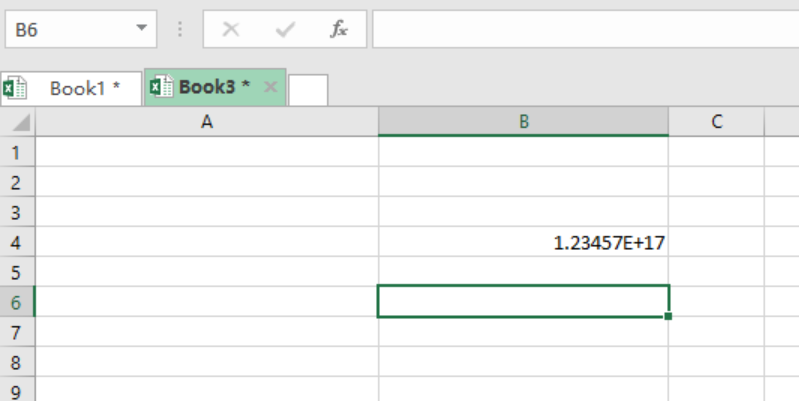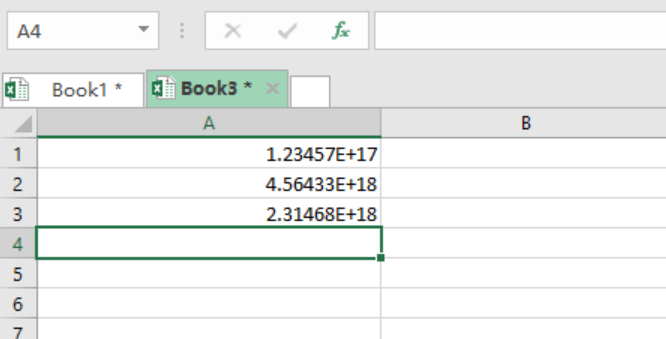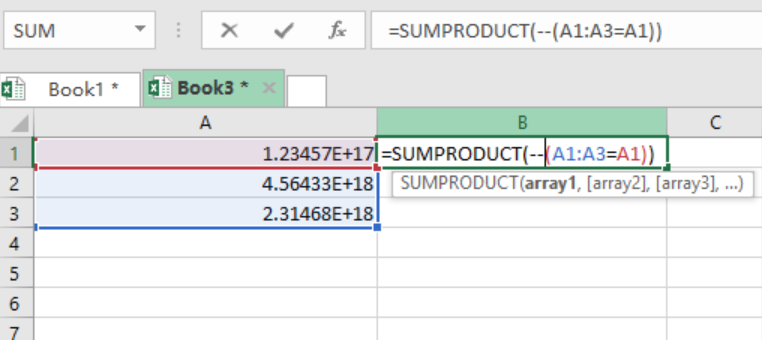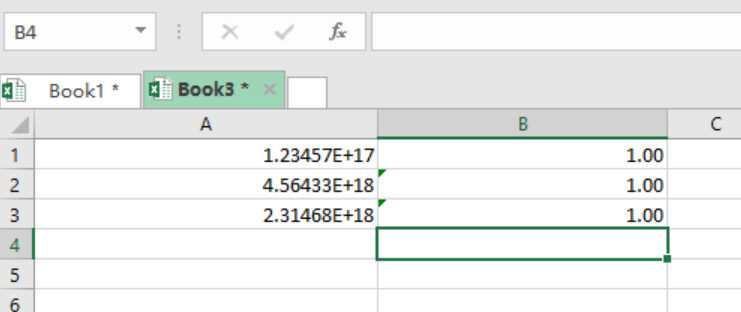Get instant live expert help with Excel or Google Sheets“My Excelchat expert helped me in less than 20 minutes, saving me what would have been 5 hours of work!”

#### Post your problem and you'll get expert help in seconds

Your message must be at least 40 characters
Our professional experts are available now. Your privacy is guaranteed.

# How to count long numbers without COUNTIF

We may want to count numbers that are greater than 15 digits. Note that Excel will return an erroneous result if we try to compute long digits with COUNTIF. This article provides a step by step guide on how to count long numbers without COUNTIF.

## Formula

`SUMPRODUCT(--(A:A=A1) `

or

`{SUM(--(A:A=A1))}`

## Explanation

SUMPRODUCT- This can be replaced by the SUM function. Its work is to do the computation.

Double negation (–) – it coerces the TRUE/FALSE result in the SUMPRODUCT computation to 1/0.

A:A– refers to the named range.eg, B10:B70

A1– specifies each cell where count should be computed.

NOTE: When we try to count long numbers that are greater than 16 in excel, we will get wrong results. This is because excel will add a trail of zeros, making it hard to use them in any computation.

## Example 1

Let us look at how excel erroneously handles digits that are greater than 16 when we use COUNTIF function.Figure 1: Showing 18 digits number in its standard form.

## Example 2

If we have a list of numbers, and want to count the number of digits in each number, we proceed as follows:

Step 1: Have the dataFigure 2: Showing the data table

Step 2: Enter the SUMPRODUCT formulaFigure 3: Showing the SUMPRODUCT formula in action

Step 3: Press Enter to get the count value as shown below:Figure 4: Showing the count values

## Notes

If we are entering extra-long numbers for non-maths operations, we can start the digits with a text. This will let us enter very many digits, without limitations.

Using COUNTIF to count numbers with digits that are more than 15 will lead to unreliable results.

## Instant Connection to an Expert through our Excelchat Service

Most of the time, the problem you will need to solve will be more complex than a simple application of a formula or function. If you want to save hours of research and frustration, try our live Excelchat service! Our Excel Experts are available 24/7 to answer any Excel question you may have. We guarantee a connection within 30 seconds and a customized solution within 20 minutes.

### Did this post not answer your question? Get a solution from connecting with the expert.Another blog reader asked this question today on Excelchat:## Subscribe to Excelchat.coAnother blog reader asked this question today on Excelchat: